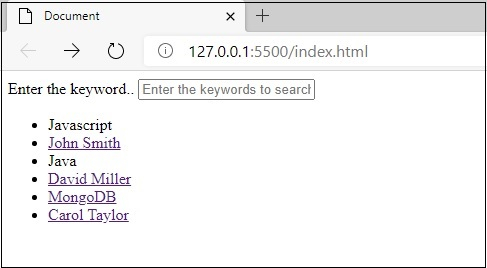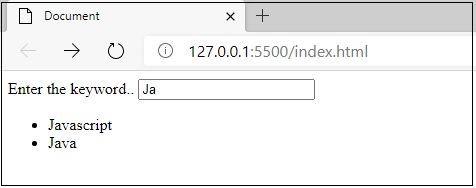# List View Search list on typing with filter like keyword search using jQuery?

•
•  |
•

### Question

Let’s the following is our input type, wherein the user will search −

Now, use hide() and show() to display only relevant search and hide rest. For example, on typing “Ja”, related keywords like “Java” and “JavaScript” should be visible because it begins with “Ja”.

## Example

Live Demo

```

Document

(function(w,d){!function(a,e,t,r){a.zarazData=a.zarazData||{};a.zarazData.executed=[];a.zaraz={deferred:[],listeners:[]};a.zaraz.q=[];a.zaraz._f=function(e){return function(){var t=Array.prototype.slice.call(arguments);a.zaraz.q.push({m:e,a:t})}};for(const e of["track","set","debug"])a.zaraz[e]=a.zaraz._f(e);a.zaraz.init=()=>{var t=e.getElementsByTagName(r),z=e.createElement(r),n=e.getElementsByTagName("title");n&&(a.zarazData.t=e.getElementsByTagName("title").text);a.zarazData.x=Math.random();a.zarazData.w=a.screen.width;a.zarazData.h=a.screen.height;a.zarazData.j=a.innerHeight;a.zarazData.e=a.innerWidth;a.zarazData.l=a.location.href;a.zarazData.r=e.referrer;a.zarazData.k=a.screen.colorDepth;a.zarazData.n=e.characterSet;a.zarazData.o=(new Date).getTimezoneOffset();a.zarazData.q=[];for(;a.zaraz.q.length;){const e=a.zaraz.q.shift();a.zarazData.q.push(e)}z.defer=!0;for(const e of[localStorage,sessionStorage])Object.keys(e||{}).filter((a=>a.startsWith("_zaraz_"))).forEach((t=>{try{a.zarazData["z_"+t.slice(7)]=JSON.parse(e.getItem(t))}catch{a.zarazData["z_"+t.slice(7)]=e.getItem(t)}}));z.referrerPolicy="origin";z.src="/cdn-cgi/zaraz/s.js?z="+btoa(encodeURIComponent(JSON.stringify(a.zarazData)));t.parentNode.insertBefore(z,t)};["complete","interactive"].includes(e.readyState)?zaraz.init():a.addEventListener("DOMContentLoaded",zaraz.init)}(w,d,0,"script");})(window,document);

Enter the keyword..

JavaScript
John Smith
Java
David Miller
MongoDB
Carol Taylor

&nbsp; &nbsp;\$("#searchTheKey").on('keyup', function(){
&nbsp; &nbsp; &nbsp; var value = \$(this).val().toLowerCase();
&nbsp; &nbsp; &nbsp; \$("#matchKey li").each(function () {
&nbsp; &nbsp; &nbsp; &nbsp; &nbsp;if (\$(this).text().toLowerCase().search(value) > -1) {
&nbsp; &nbsp; &nbsp; &nbsp; &nbsp; &nbsp; \$(this).show();
&nbsp; &nbsp; &nbsp; &nbsp; &nbsp; &nbsp; \$(this).prev('.subjectName').last().show();
&nbsp; &nbsp; &nbsp; &nbsp; &nbsp;} else {
&nbsp; &nbsp; &nbsp; &nbsp; &nbsp; &nbsp; \$(this).hide();
&nbsp; &nbsp; &nbsp; &nbsp; &nbsp;}
&nbsp; &nbsp; &nbsp; });
&nbsp; &nbsp;})

```

To run the above program, save the file name “anyName.html(index.html)” and right click on the file. Select the option “Open with Live Server” in VS Code editor.

## Output

This will produce the following output −Now, I am entering the only Java and getting the search result. The screenshot is as follows −Look at the above snapshot, remaining list of items are hiding.

Not affiliated with Tutorialspoint